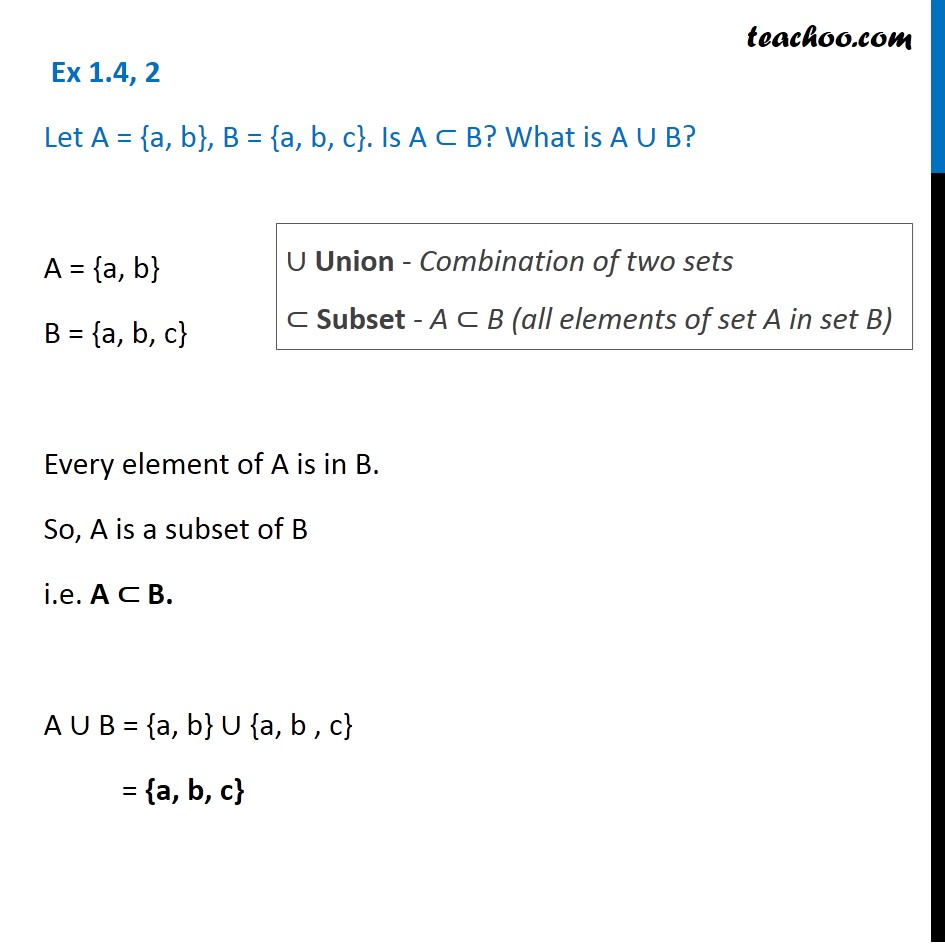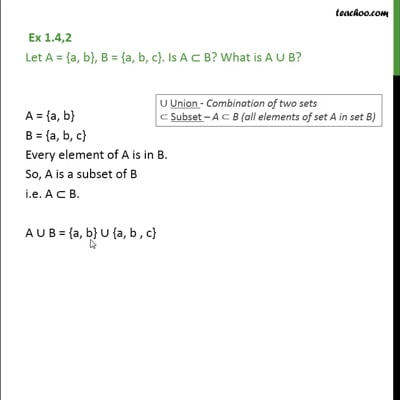Ex 1.4

Chapter 1 Class 11 Sets (Term 1)
Serial order wiseThis video is only available for Teachoo black users

### Transcript

Ex 1.4,2 Let A = {a, b}, B = {a, b, c}. Is A B? What is A B? A = {a, b} B = {a, b, c} Every element of A is in B. So, A is a subset of B i.e. A B. A B = {a, b} {a, b , c} = {a, b, c}